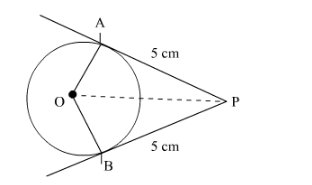# Prove that the lengths of tangents drawn`
Question:

Prove that the lengths of tangents drawn from an external point to a circle are equal.

Solution:

We have to prove that the lengths of tangents drawn from an external point to a circle are equal.Draw a circle with centre O and tangents PA and PB, where P is the external point and and B are the points of contact of the tangents.
Join OAOB and OP.

Now in $\triangle O A P$ and $\triangle O B P$

$\angle O A P=\angle O B P \quad\left[\right.$ Both $90^{\circ}$, because radius is perpendicular to the tangent $] .$

$O P=O P$   [common]

$O A=O B$   [radii]

Therefore $\Delta \mathrm{OAP} \cong \Delta \mathrm{OBP}$ [RHS congruency]

$P A=P B \quad$ (Congruent property of congruent triangles)

Hence proved.